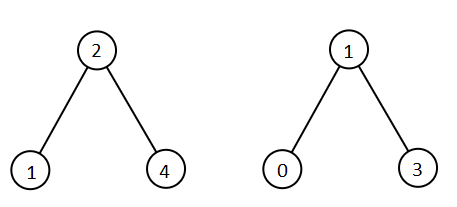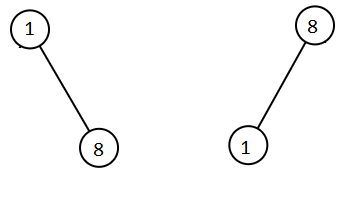# 两棵二叉搜索树中的所有元素

## 1305. 两棵二叉搜索树中的所有元素 (Medium)```输入：root1 = [2,1,4], root2 = [1,0,3]

```

```输入：root1 = [0,-10,10], root2 = [5,1,7,0,2]

```

```输入：root1 = [], root2 = [5,1,7,0,2]

```

```输入：root1 = [0,-10,10], root2 = []

``````输入：root1 = [1,null,8], root2 = [8,1]

```

• 每棵树最多有 `5000` 个节点。
• 每个节点的值在 `[-10^5, 10^5]` 之间。

### 相关话题

[] [深度优先搜索] [二叉搜索树] [二叉树] [排序]